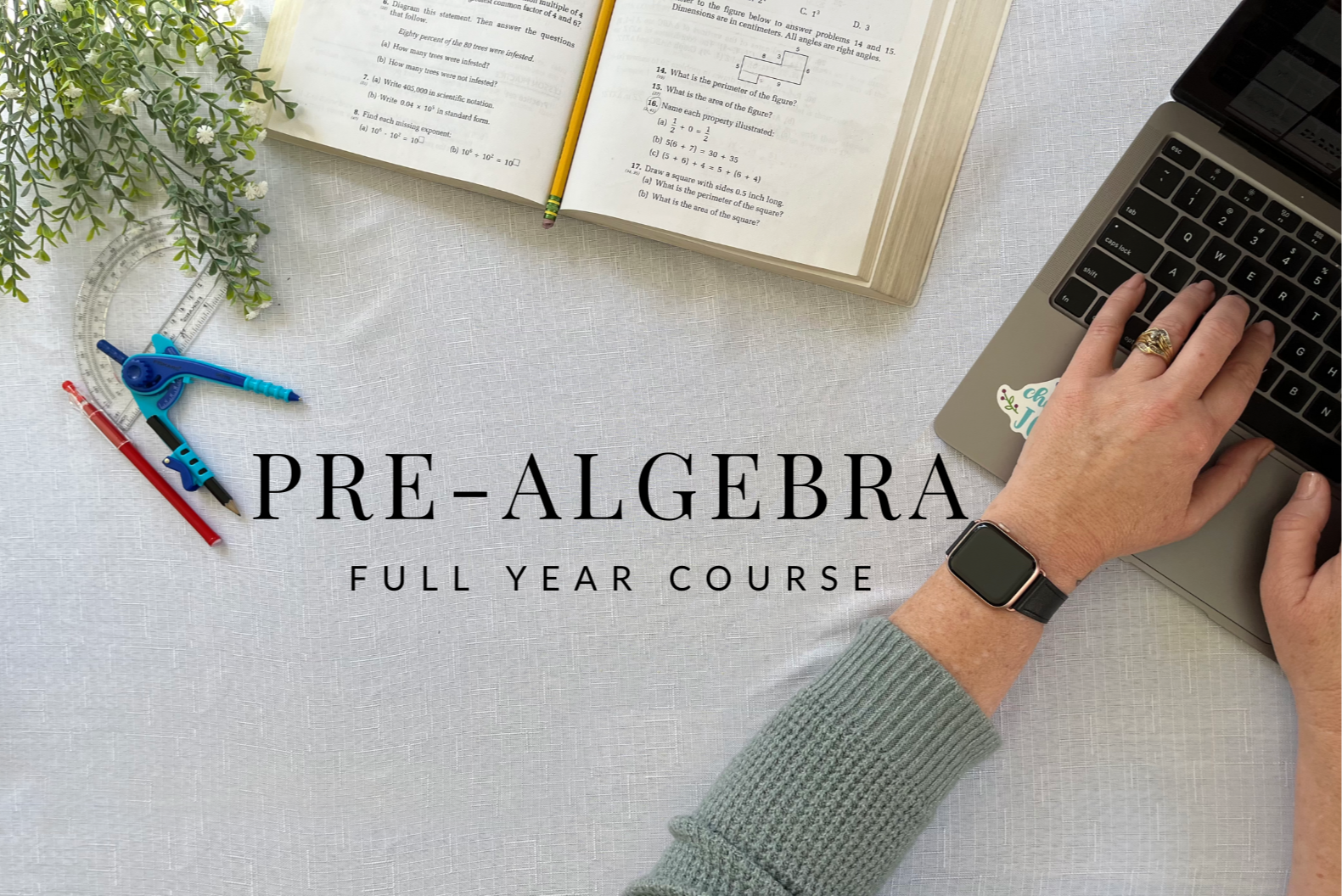## Algebra I

Algebra I is a crucial course that provides students with a deep understanding of essential algebraic concepts, including variables, equations, inequalities, functions, graphs, and more.  Mastering Algebra I not only develops critical thinking, problem-solving, and analytical skills, but also lays a solid foundation for future math and science courses such as geometry, trigonometry, physics, and calculus.## pre-Algebra

Pre-algebra is an essential and exciting course that lays the foundation for more advanced math concepts. It introduces students to the fundamental principles of mathematics, such as operations with integers, fractions, decimals, basic equations, and more. Pre-Algebra teaches critical reasoning, logical thinking, and problem-solving strategies, These are life skills that will help students tackle complex problems, make informed decisions, and communicate effectively.